# Style sheets reference¶

This script demonstrates the different available style sheets on a common set of example plots: scatter plot, image, bar graph, patches, line plot and histogram,

•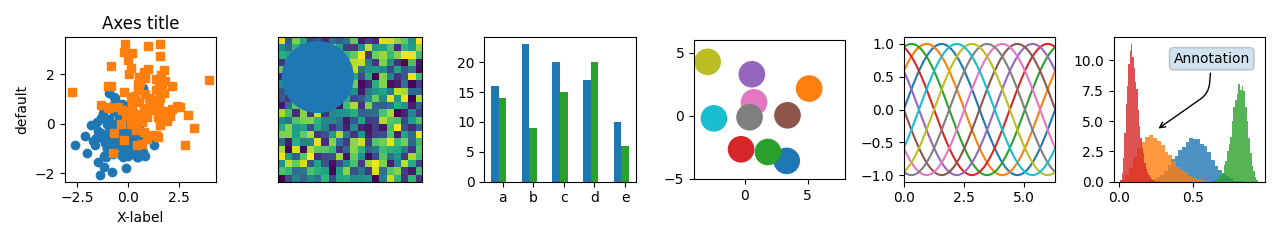•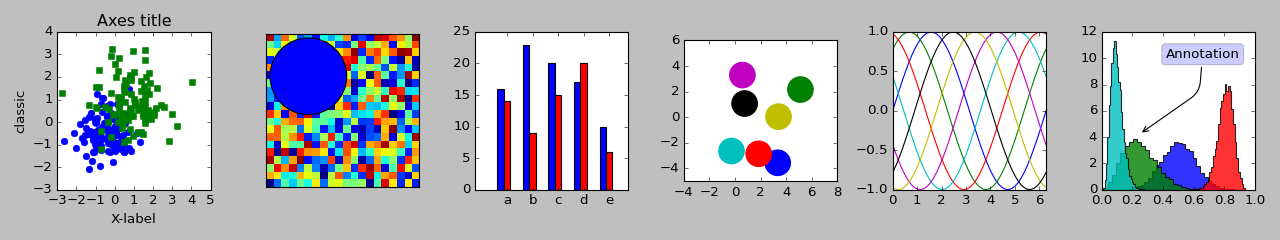•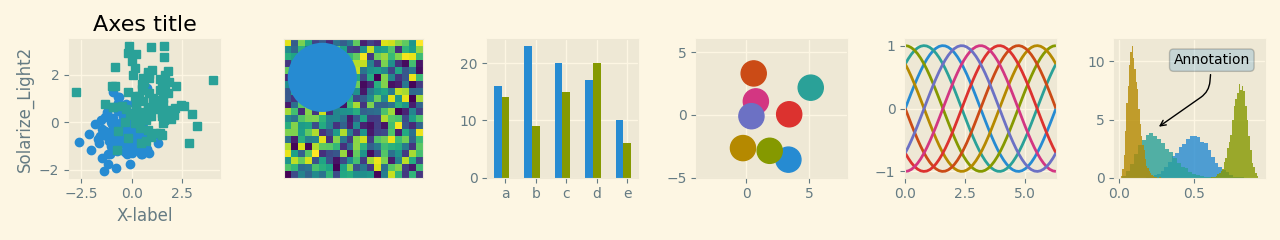•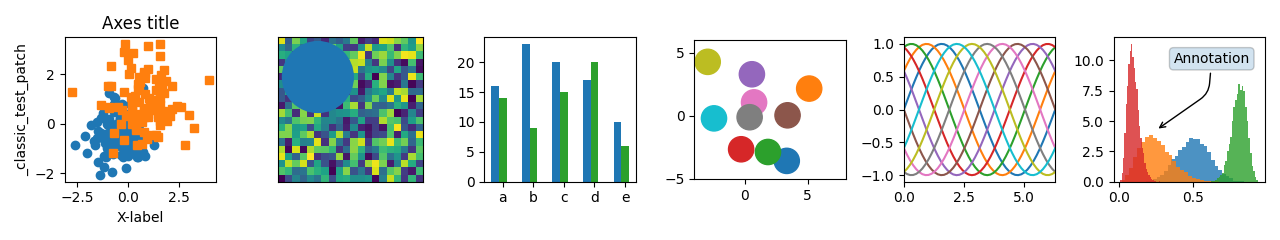•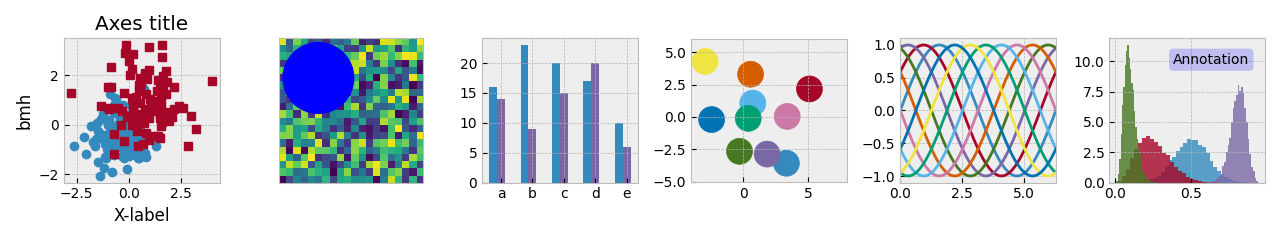•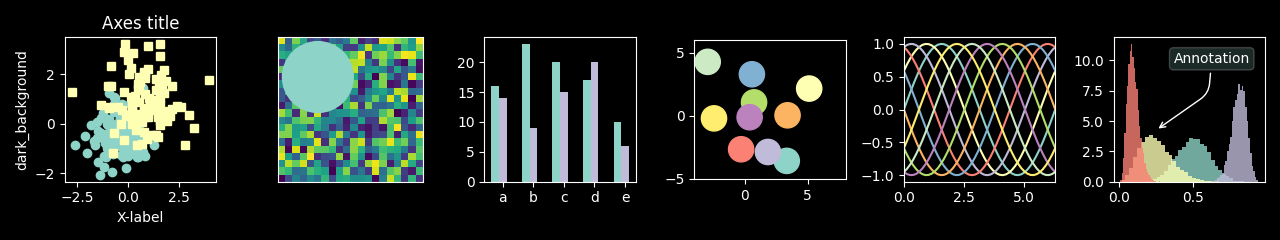•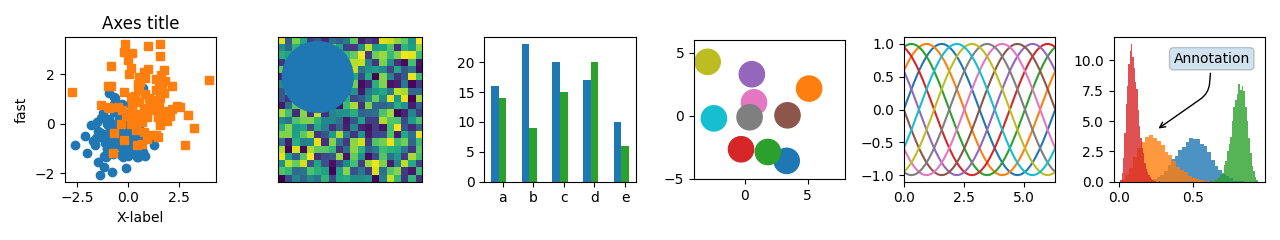•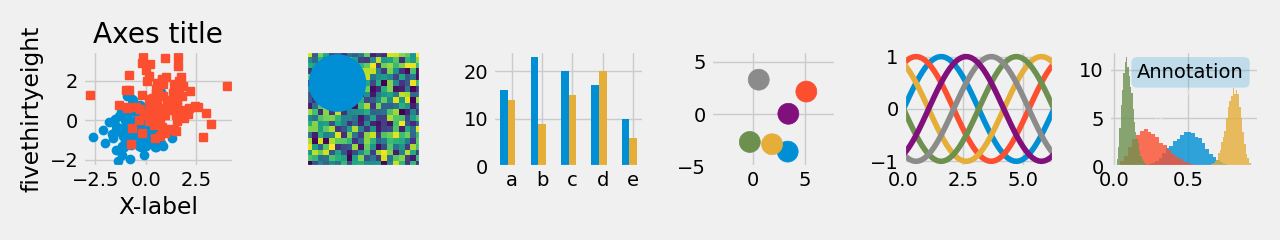•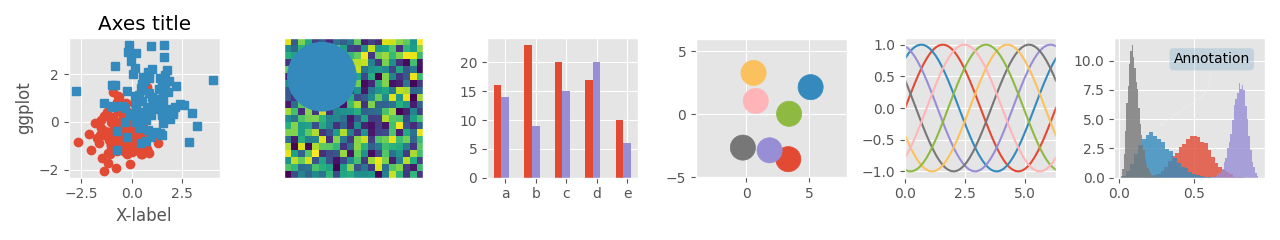•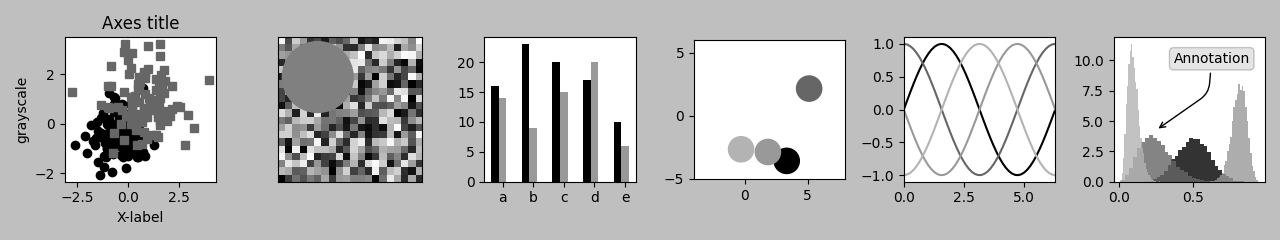•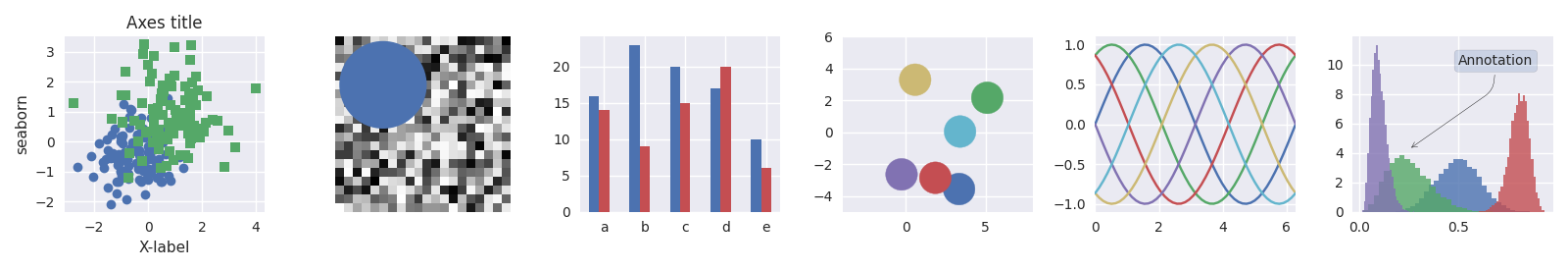•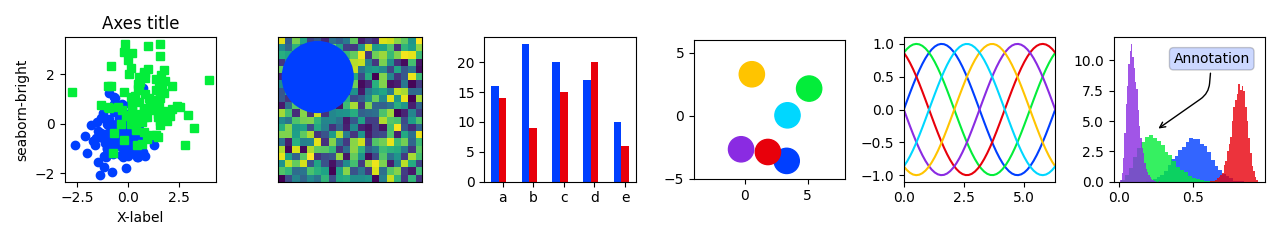•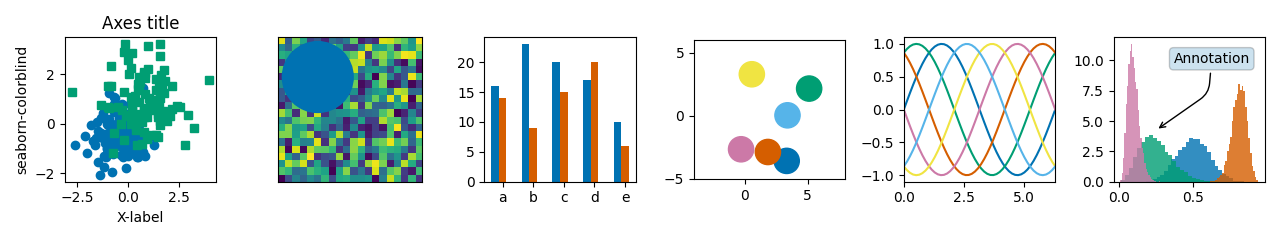•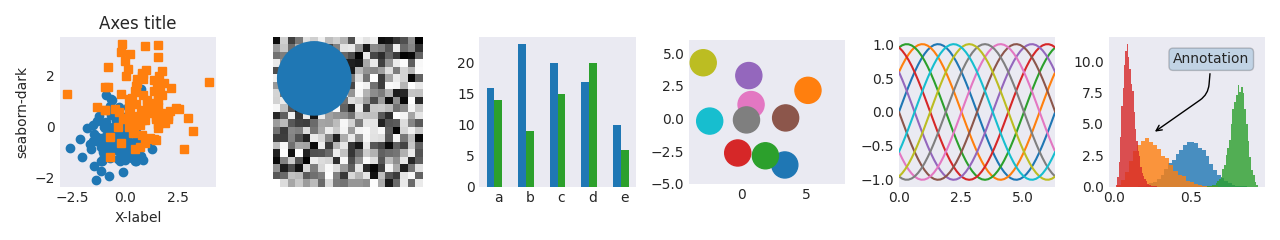•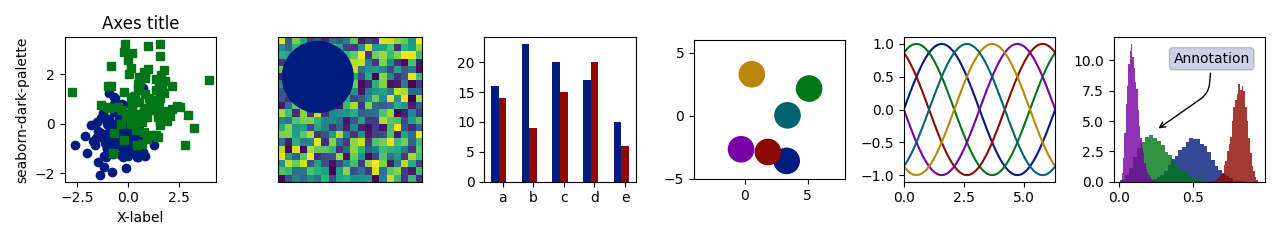•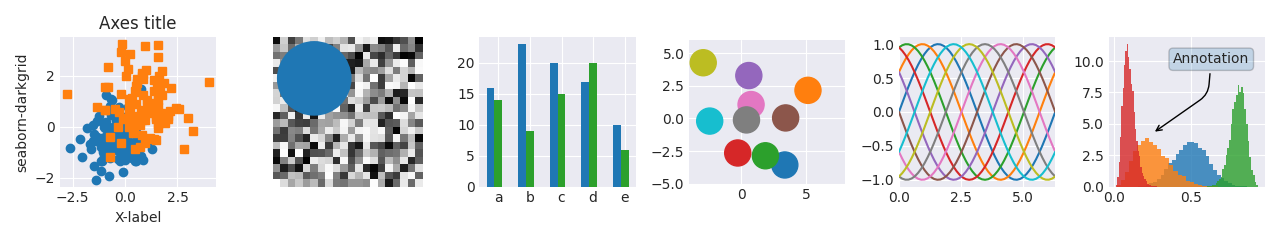•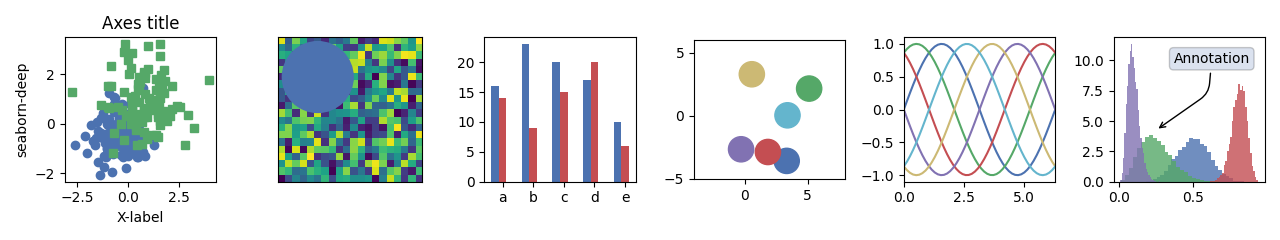•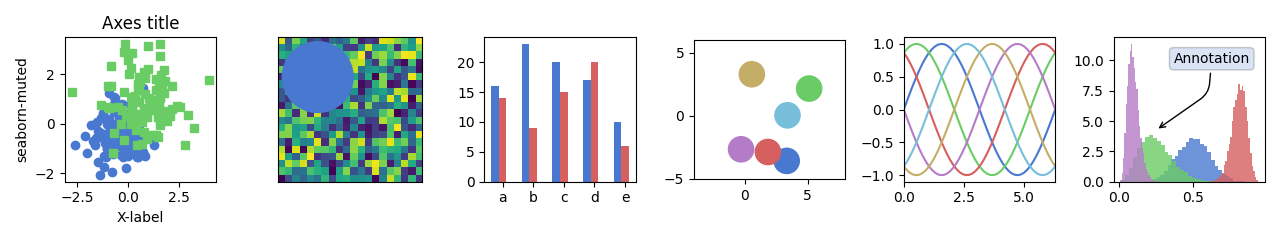•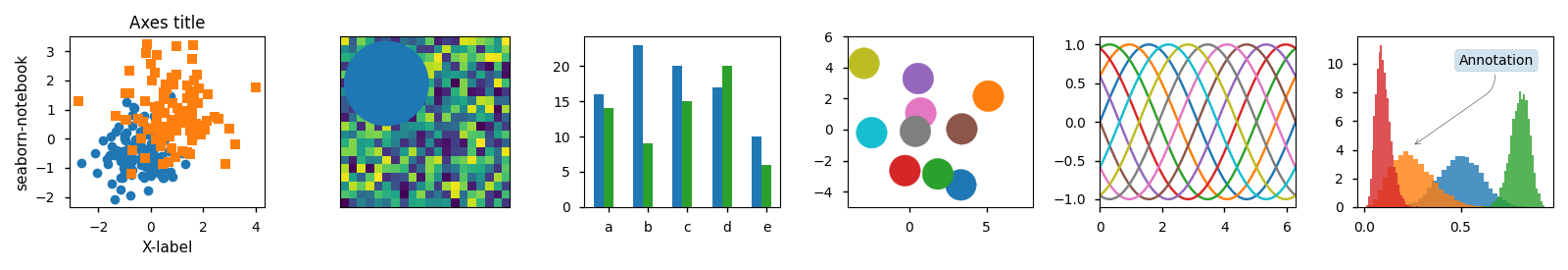•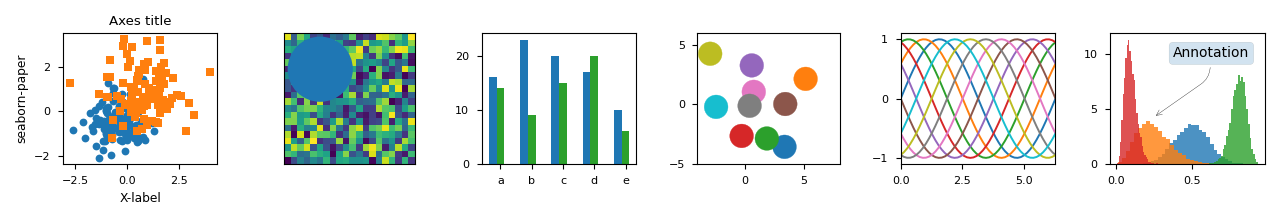•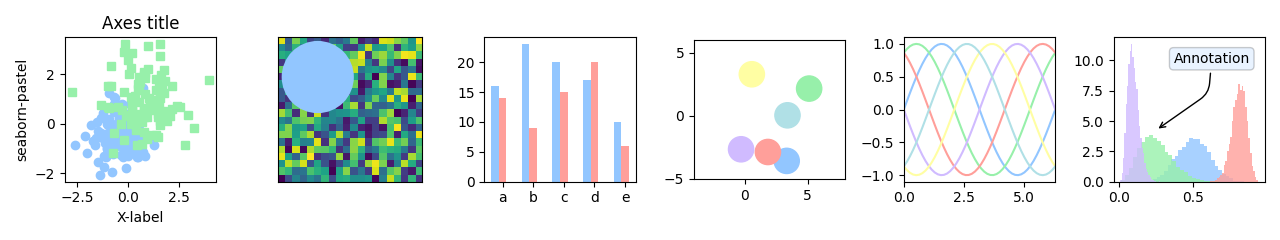•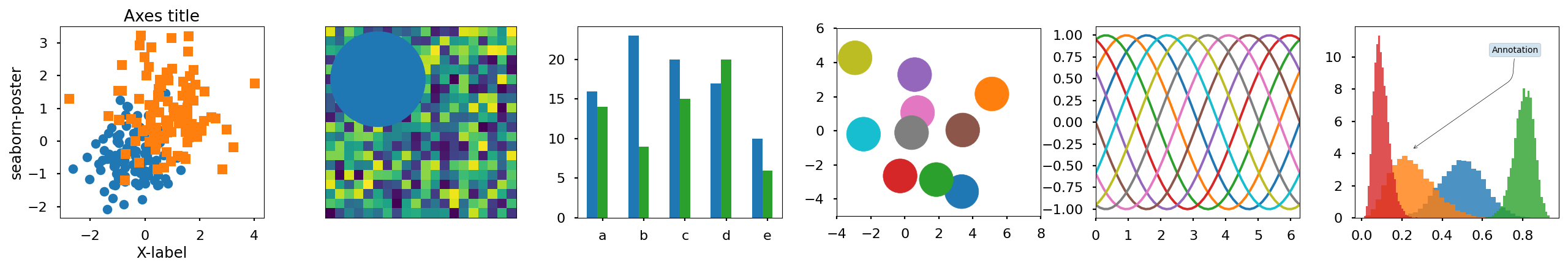•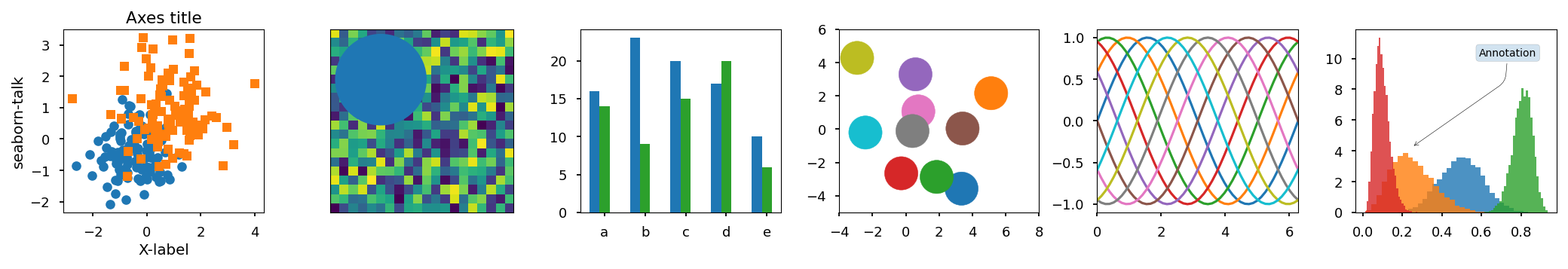•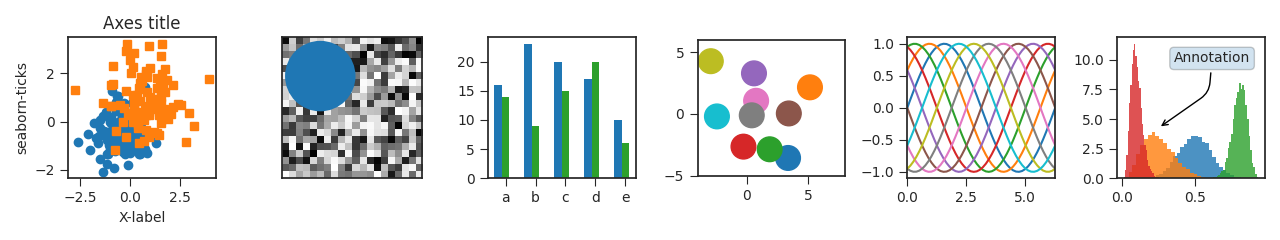•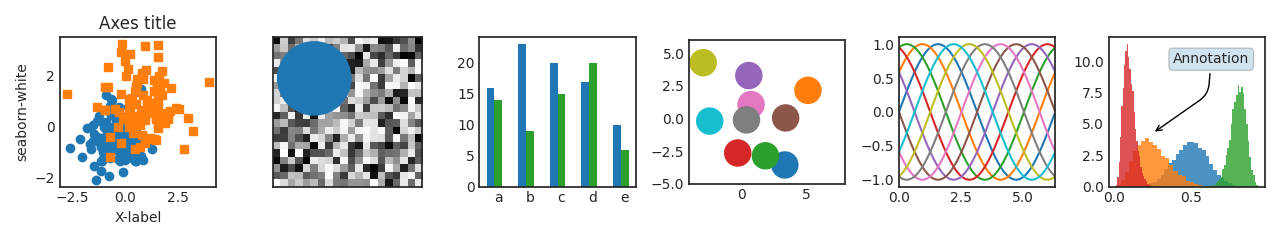•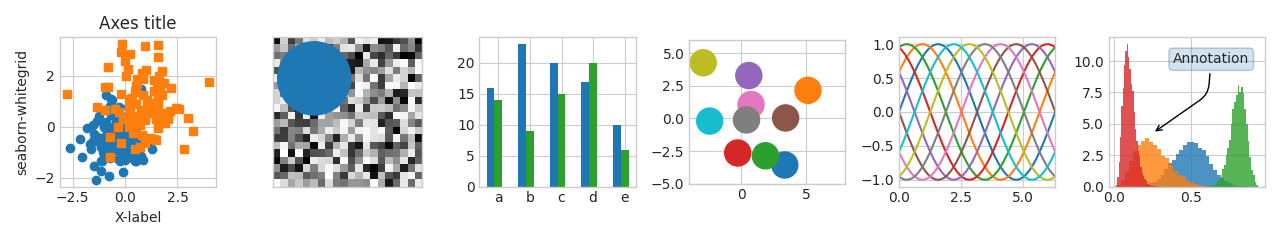•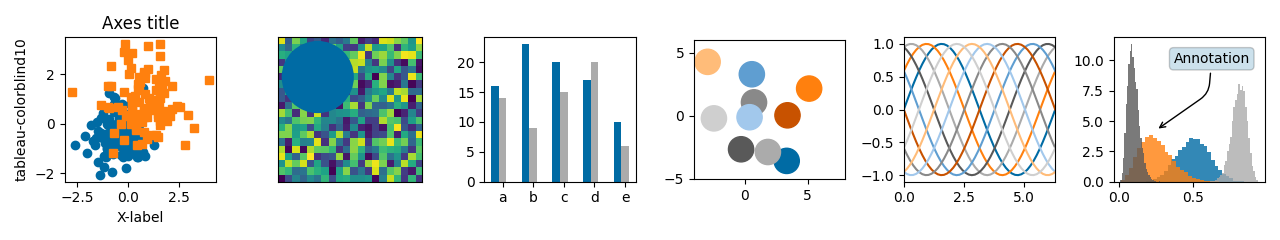Out:

/matplotlib/examples/style_sheets/style_sheets_reference.py:114: RuntimeWarning: More than 20 figures have been opened. Figures created through the pyplot interface (matplotlib.pyplot.figure) are retained until explicitly closed and may consume too much memory. (To control this warning, see the rcParam figure.max_open_warning).
fig, axs = plt.subplots(ncols=6, nrows=1, num=style_label,


import numpy as np
import matplotlib.pyplot as plt

# Fixing random state for reproducibility
np.random.seed(19680801)

def plot_scatter(ax, prng, nb_samples=100):
"""Scatter plot."""
for mu, sigma, marker in [(-.5, 0.75, 'o'), (0.75, 1., 's')]:
x, y = prng.normal(loc=mu, scale=sigma, size=(2, nb_samples))
ax.plot(x, y, ls='none', marker=marker)
ax.set_xlabel('X-label')
ax.set_title('Axes title')
return ax

def plot_colored_sinusoidal_lines(ax):
"""Plot sinusoidal lines with colors following the style color cycle."""
L = 2 * np.pi
x = np.linspace(0, L)
nb_colors = len(plt.rcParams['axes.prop_cycle'])
shift = np.linspace(0, L, nb_colors, endpoint=False)
for s in shift:
ax.plot(x, np.sin(x + s), '-')
ax.set_xlim([x, x[-1]])
return ax

def plot_bar_graphs(ax, prng, min_value=5, max_value=25, nb_samples=5):
"""Plot two bar graphs side by side, with letters as x-tick labels."""
x = np.arange(nb_samples)
ya, yb = prng.randint(min_value, max_value, size=(2, nb_samples))
width = 0.25
ax.bar(x, ya, width)
ax.bar(x + width, yb, width, color='C2')
ax.set_xticks(x + width)
ax.set_xticklabels(['a', 'b', 'c', 'd', 'e'])
return ax

def plot_colored_circles(ax, prng, nb_samples=15):
"""
Plot circle patches.

NB: draws a fixed amount of samples, rather than using the length of
the color cycle, because different styles may have different numbers
of colors.
"""
for sty_dict, j in zip(plt.rcParams['axes.prop_cycle'], range(nb_samples)):
# Force the limits to be the same across the styles (because different
# styles may have different numbers of available colors).
ax.set_xlim([-4, 8])
ax.set_ylim([-5, 6])
ax.set_aspect('equal', adjustable='box')  # to plot circles as circles
return ax

def plot_image_and_patch(ax, prng, size=(20, 20)):
"""Plot an image with random values and superimpose a circular patch."""
values = prng.random_sample(size=size)
ax.imshow(values, interpolation='none')
c = plt.Circle((5, 5), radius=5, label='patch')
# Remove ticks
ax.set_xticks([])
ax.set_yticks([])

def plot_histograms(ax, prng, nb_samples=10000):
"""Plot 4 histograms and a text annotation."""
params = ((10, 10), (4, 12), (50, 12), (6, 55))
for a, b in params:
values = prng.beta(a, b, size=nb_samples)
ax.hist(values, histtype="stepfilled", bins=30,
alpha=0.8, density=True)
ax.annotate('Annotation', xy=(0.25, 4.25),
xytext=(0.9, 0.9), textcoords=ax.transAxes,
va="top", ha="right",
bbox=dict(boxstyle="round", alpha=0.2),
arrowprops=dict(
arrowstyle="->",
)
return ax

def plot_figure(style_label=""):
"""Setup and plot the demonstration figure with a given style."""
# Use a dedicated RandomState instance to draw the same "random" values
# across the different figures.
prng = np.random.RandomState(96917002)

# Tweak the figure size to be better suited for a row of numerous plots:
# double the width and halve the height. NB: use relative changes because
# some styles may have a figure size different from the default one.
(fig_width, fig_height) = plt.rcParams['figure.figsize']
fig_size = [fig_width * 2, fig_height / 2]

fig, axs = plt.subplots(ncols=6, nrows=1, num=style_label,
figsize=fig_size, squeeze=True)
axs.set_ylabel(style_label)

plot_scatter(axs, prng)
plot_image_and_patch(axs, prng)
plot_bar_graphs(axs, prng)
plot_colored_circles(axs, prng)
plot_colored_sinusoidal_lines(axs)
plot_histograms(axs, prng)

fig.tight_layout()

return fig

if __name__ == "__main__":

# Setup a list of all available styles, in alphabetical order but
# the default and classic ones, which will be forced resp. in
# first and second position.
style_list = ['default', 'classic'] + sorted(
style for style in plt.style.available if style != 'classic')

# Plot a demonstration figure for every available style sheet.
for style_label in style_list:
with plt.style.context(style_label):
fig = plot_figure(style_label=style_label)

plt.show()


Total running time of the script: ( 0 minutes 18.651 seconds)

Keywords: matplotlib code example, codex, python plot, pyplot Gallery generated by Sphinx-Gallery